Your data analysis solution

# Simulation model with scenario variables tutorial

2017-10-20

This tutorial will help you set up and run a simulation model that includes a scenario variable in Excel using the XLSTAT statistical software.

## Simulation models

Simulation models allow to obtain information, such as mean or median or confidence intervals, on variables that do not have an exact value, but for which we either know or assume a distribution. If some “result” variables depend on these “distributed” variables by the way of known or assumed formulae, then the “result” variables will also have a distribution. Sim allows you to define the distributions, and then to obtain, through simulations, an empirical distribution of the input and output variables as well as the corresponding statistics.

Simulation models are used in many areas such as finance and insurance, medicine, oil and gas prospecting, accounting, or sales prediction.

Four elements are involved in the construction of a simulation model:

1. Distributions are associated to random variables. XLSTAT gives a choice of more than 30 distributions to describe the uncertainty on the values that a variable can take. For example, you can choose a triangular distribution if you have a quantity for which you know it can vary between two bounds, but with a value that is more likely (a mode). At each iteration of the computation of the simulation model, a random draw is performed for each distribution that has been defined.
2. Scenario variables allow to include in the simulation model a quantity that is fixed in the model, except during the tornado analysis where it can vary between two bounds.
3. Result variables correspond to outputs of the model. They depend either directly or indirectly, through one or more Excel formulae, on the random variables to which distributions have been associated and, if available, on the scenario variables. The goal of computing the simulation model is to obtain the distribution of the result variables.
4. Statistics allow to track a given statistic for a result variable. For example, we might want to monitor the standard deviation of a result variable.

A correct model should comprise at least one distribution and one result variable. Models can contain any number of these four elements. A model can be limited to a single Excel sheet or can use a whole Excel folder.

## Dataset to run a simulation including scenario variables and statistics

An Excel sheet containing both the data and the results for use in this tutorial can be downloaded by clicking here.

In this tutorial, the same model as in the first tutorial is used. It is extended with a scenario variable and a statistic.

Our simulation model is based on sales and costs of a shop. The benefit is simply the difference between sales and costs in this simple case. Based on historical data for costs and sales that were analyzed with the tool distribution fitting we found out that the costs follow a normal distribution (mu=120, sigma=10) and the sales a normal distribution (mu=80, sigma=20) (see tutorial on distribution fitting for more details).

Additionally we suppose that 80 % of the costs and 30 % of the sales depend on a currency exchange rate. The exchange rate is set to 1 at the beginning. During the tornado analysis, several scenarios of an exchange rate from 0.8 to 1.2 are simulated.

Sales and costs are defined as in tutorial sim1. The formula of the benefit is more complicated because we now take into account the exchange rate: = (0.3 * E2 * B2 + 0.7 *B2) -( 0.8 * E2* B3+ 0.2 * B3).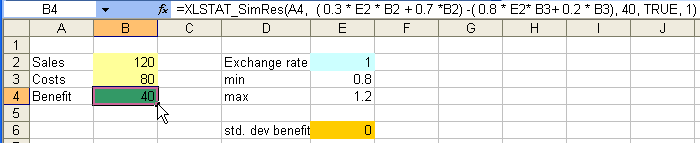The scenario variable and the statistic will now be added to the model that was created on the "Model" sheet. Select the E2 cell that has the value of the exchange rate as active cell. The scenario variable is going to be defined there.

## Setting up a scenario variable

Once XLSTAT is activated, select the XLSTAT / Sim / Define a scenario variable command, or click the corresponding button of the Sim toolbar (see below).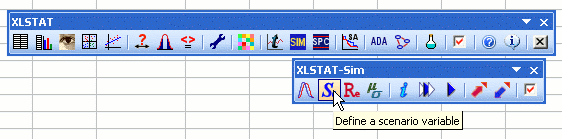The Define a scenario variable dialog box appears. Select the Excel cell that contains “exchange rate” to define the name of the variable.

Select the cell with the value 0.8 for the lower limit and for the upper limit the cell with the value 1.2. This defines the limits between which the exchange rate varies.

Choose the continuous data type, as the exchange rate can take every value between 0.8 and 1.2.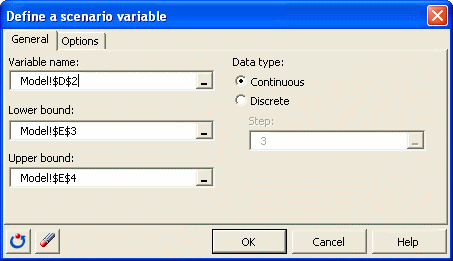Once you have clicked on OK, the corresponding function call of XLSTAT_SimSVar is inserted into the active cell.

## Defining a statistic

Select the result cell that is to the right of the cell containing “std. dev benefit” to define a statistic and track the standard deviation of the result variable "benefit" during the simulation run. This statistic will be calculated at each simulation iteration. Select the XLSTAT / Sim / Define a statistic command, or on the corresponding button of the Sim toolbar.

The Define a statistic dialog box appears. Select the Excel cell with the name “std. dev benefit” (D6). Activate the option Descriptive statistics and select standard deviation (n-1) in the list.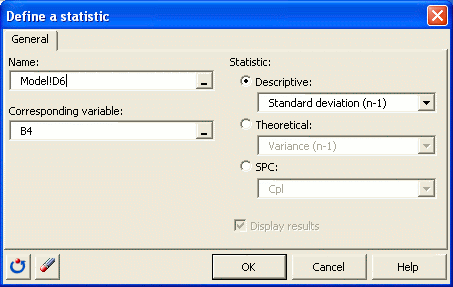Once you have clicked on OK, the corresponding function call to XLSTAT_SimStat is inserted into the active cell (E6).

## Running a simulation with scenario variables and statistics

Sim allows you to do the simulation in step by step mode, if you prefer to do so. This allows you to verify that your model behaves as expected. In this example you are able to track the evolution of the defined statistic step by step.

To initialize the simulation model and to delete the information saved from an earlier step by step simulation, click on the XLSTAT / Sim / Reinitialize the simulation model command, or click the corresponding button of the Sim toolbar.

You will see the results of the first simulation iteration in the cells corresponding to "sales" , "costs" and "benefit". The scenario variable will only be used during the tornado analysis and does not change its value for now. The statistic is not yet calculated, because there have to be at least two historical values to calculate the standard deviation.

To do further simulation steps, select the XLSTAT / Sim / Do a simulation step command, or click the corresponding button of the Sim toolbar.

You can see how the statistic of the standard deviation rapidly converges.

To start the simulation run, select the XLSTAT / Sim / Simulation - Run command, or click the corresponding button of the Sim toolbar.

The Simulation - Run dialog box appears. Set the number of simulations to 1000.In the Options tab, enter the parameters of the tornado and spider analysis.

Select the standard cell value as default value. Choose 10 data points in the interval from -10% up to +10% of the value deviation: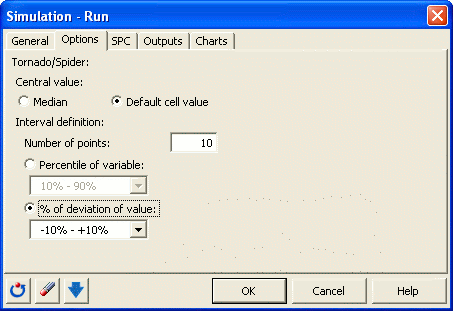In the Outputs tab, we activate the option Simulation details.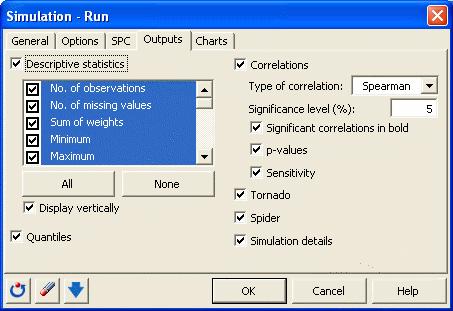The computations begin once you have clicked OK.

## Interpreting the results of a simulation model with scenario variables and statistics

The first results are the same as the ones described in the first tutorial.

Tornado and spider analyses are not based on the iterations of the simulation but on a point by point analysis of all the input variables (random variables with distributions and scenario variables).

During the tornado analysis, for each result variable, each input random variable and each scenario variable are studied one by one. We make their value vary between two bounds and record the value of the result variable in order to know how each random and scenario variable impacts the result variables. For a random variable, the values explored can either be around the median or around the default cell value, with bounds defined by percentiles or deviation. For a scenario variable, the analysis is performed between the two bounds specified when defining the variables. The number of points is an option that can be modified by the user before running the simulation model.

In the tornado analysis, the results for the scenario variable are displayed. Its impact is on the same level of importance as the two other variables "costs" and "sales". Further details are visible in the spider diagram. The exchange rate is clearly negatively correlated. A rising exchange rate shrinks the benefit.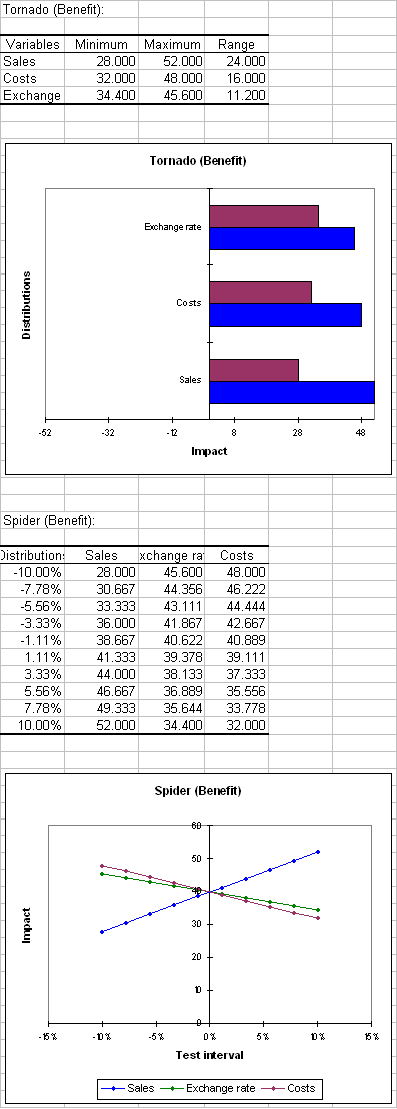Finally the simulation details are displayed. For each of the model variables "costs", "sales", "benefit", and "std. dev benefit" the evolution at each iteration is displayed. We see clearly the evolution of the statistic and conclude that the statistic quickly converges towards 22 and then only oscillates around this value.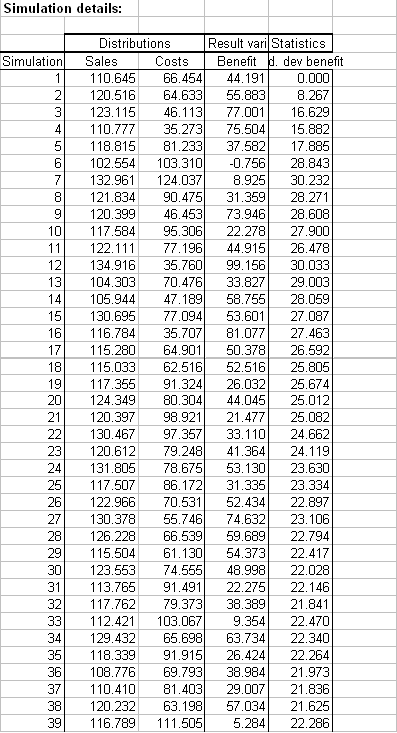### Contact Us

#### Contact our technical support team: support@xlstat.com

https://cdn.desk.com/
false
desk
Loading
seconds ago
a minute ago
minutes ago
an hour ago
hours ago
a day ago
days ago
about
false
Invalid characters found
/customer/portal/articles/autocomplete
9283